Next: Appendix B: Tito Livio Up: Why does the meter Previous: Acknowledgements

# Appendix A: The local meter' and second' in the planets of the solar system

The well known small angle formula that gives the period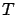of the simple pendulum (i.e. the elementary text book pendulum) as a function of its length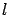is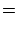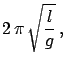(1)

where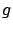is the gravitational acceleration, approximately equal to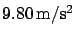on Earth. For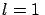m we gets. Therefore, each swing takes 1.0035s, that differs from a round second only by a few parts per thousand. Varyingby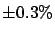(i.e. fromto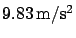), the period changes only by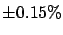.

In order to understand if there is any physical reason behind this numerical coincidence let us try to understand the property of Earth that mainly influences the period of the pendulum, and if there is any simplification due to the fact that the length of the pendulum is about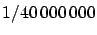of the meridian.44 The gross value ofdepends on mass and radius45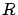of the Earth with local effects due to not exact sphericity (see Table 4), mass dishomogeneity and above sea level height. Moreover, there is a centrifugal term, null at the pole and maximum at the equator, due to Earth rotation.46In the approximation of a perfect sphere, the gravitational acceleration, i.e. the gravitational force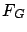divided by the mass of the pendulum, is given by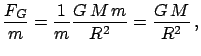(2)

where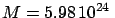kg is the mass of Earth and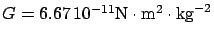is the gravitational constant. Expressing the mass in terms of density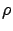and volume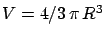, we get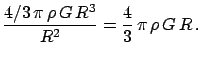(3)

The gravitational accelerationis then proportional to the planet size and density. Let us now calculate the period of a pendulum whose length ispart of a meridian of a spherical planet, i.e.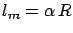, where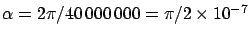is the fixed ratio between this meter' and the planet radius. The period of such a planetary meter' pendulum is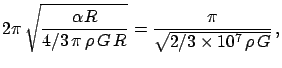(4)

and depends only on planet density, and not on planet mass and size separately. In particular, in the inner planets and Earth, for which the density is approximately 5.5 g/cm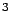, such a planetary meter' pendulum would beat approximately the second (see Tab. 6).

 Planet Physical data  One meter' pendulum and its period Mass Radius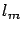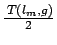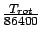(kg) (km) (g/cm) (m/s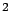) (m) (s) (s) Mercury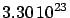2440 5.43 3.70 0.38 1.01 58.6 Venus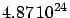6052 5.24 8.89 0.95 1.03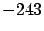Earth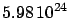6378 5.52 9.80 1.00 1.00 1.00 Mars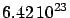3397 3.93 3.69 0.53 1.19 1.03 Jupiter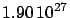71492 1.33 23.17 11.23 2.19 0.41 Saturn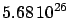60268 0.69 8.98 9.47 3.23 0.45 Uranus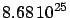25559 1.32 8.71 4.01 2.13 0.72 Neptune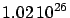24766 1.64 11.03 3.89 1.87 0.67 Pluto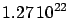1137 2.06 0.66 0.19 1.64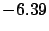However, the half period of this pendulum is approximately equal to the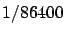part of the planet rotation only for Earth and Mars, which have approximately equal `days'. For all other planets, the local day can be very different with respect to the Earth one. In fact, the rotation speed is related to the initial angular momentum when the planet was formed and there is no reason why it should come out to be the same in different planets (Venus and Pluto are indeed retrograde, i.e. they rotate East-West).Next: Appendix B: Tito Livio Up: Why does the meter Previous: Acknowledgements
Giulio D'Agostini 2005-01-25